# 大话数据结构 －－ KMP模式匹配算法

## 1、串的匹配是串的基础同时也非常重要的操作，最初人们用朴素的模式匹配算法，即：

int Index(String S, String T, int pos){    //在主串S中找到从pos开始与子串T相匹配的第一个字符的位置
int i=pos;
int j=1;
while(i<=S&&j<=T){    // 注意i<=S和j<=T要同时满足
if(S[i]==T[j]){    //匹配一致，则均后移继续匹配
i++;
j++;
}
else{        //匹配不一致，则主串下标后移一位，子串下标置1
i=i-j+2;
j=1;
}
}
if(j>T)    //j>T说明子串全部比较完且与主串中某一部分一致
return i-T;	// 注意i才是主串的下标，i-T返回开始匹配的位置
else
return 0;
}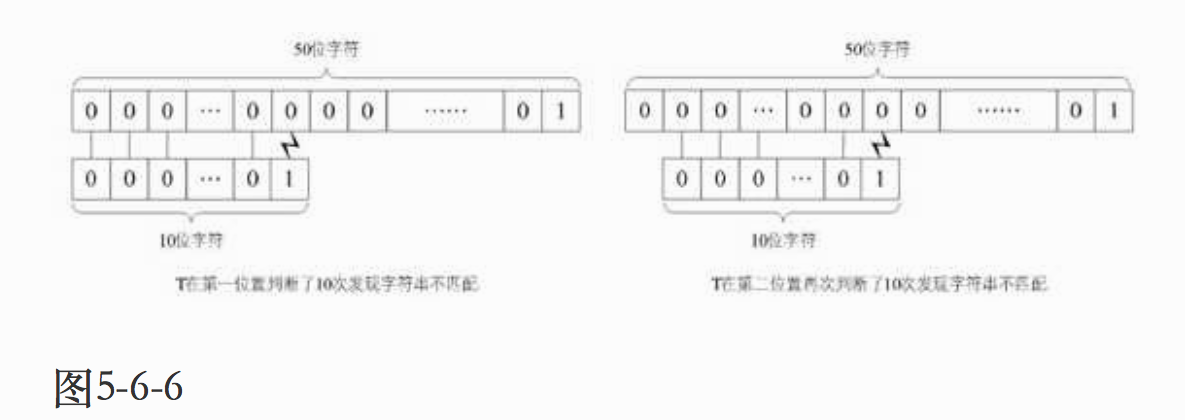## 2、KMP模式匹配算法发现了朴素算法的不足，主要特点是：

### 2）、待匹配串中存在回文相等，且它们在主串中有对应的子串，当后一位匹配失败时，待匹配串中回文串的前几位不必再与主串中明显与自己相等的字符去比较。

// 通过计算返回子串T的next数组
void get_next(String T, int *next){
int i,j;
i=1;
j=0;
next=0;
// 此处T表示串T的长度
while(i<T){
// T[i]表示后缀的单个字符
// T[j]表示前缀的单个字符
if(j==0 || T[i]==T[j]){
++i;
++j;
next[i]=j;
}
else
// 若字符不相同，则j值回溯
j=next[j];
}
}

// 返回子串T在主串S中第pos个字符之后的位置。
// 若不存在，则函数返回值为0
// T非空，1<=pos<=StrLength(S)
int Index_KMP(String S, String T, int pos){
// i 用于主串S当前位置下标值，若pos不为1，则从pos位置开始匹配
int i=pos;
//j 用于子串T中当前位置下标值
int j=1;
// 定义next数组
int next;

// 对串T作分析，得到next数组
get_next(T,next);

// 若i小于S的长度且j小于T的长度时，循环继续
while(i<=S && j<=T){
// 两字母相等则继续，相对于朴素算法增加了
// j=0 判断
if(j==0||S[i]==T[j]){
++i;
++j;
}
// 指针后退重新开始匹配
else{
// j退回合适的位置，i值不变
j=next[j];
}
}
if(j>T){
return i-T;
}
else
return 0;
}


## 3、KMP模式匹配算法的改进：

### 2）、若当前字符与前缀字符相同，则将前缀字符的nextval值赋值给nextval在i位置的值。

//KMP模式匹配算法的改进

// 求模式串T的next函数修正值并存入数组nextval
void get_nextval(String T,int *nextval){
int i,j;
i=1;
j=0;
nextval=0;
while(i<T){
if(j==0||T[i]==T[j]){
i++;
j++;
// 若当前字符与前缀字符不同
if(T[i]!=T[j])
// 则当前的j为nextval在i位置的值
nextval[i]=j;
else
//如果与前缀字符相同，则将前缀字符的nextval值赋值给nextval在i位置的值
nextval[i]=nextval[j];
}
else
//若字符不相同，则j值回溯
j=nextval[j];
}
}

－－－－－－－－－－－－－－－－－－－－－－－－－－－－－－－－－－－－－－－－－－－－－－－－－－－－－－－－

# 概念引入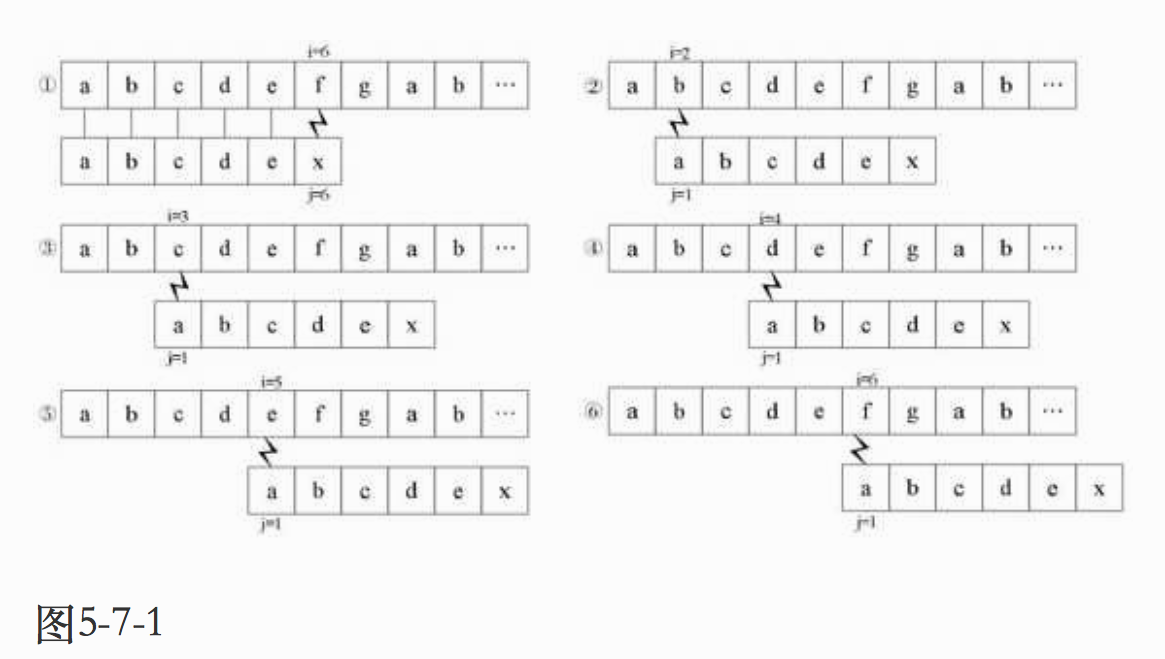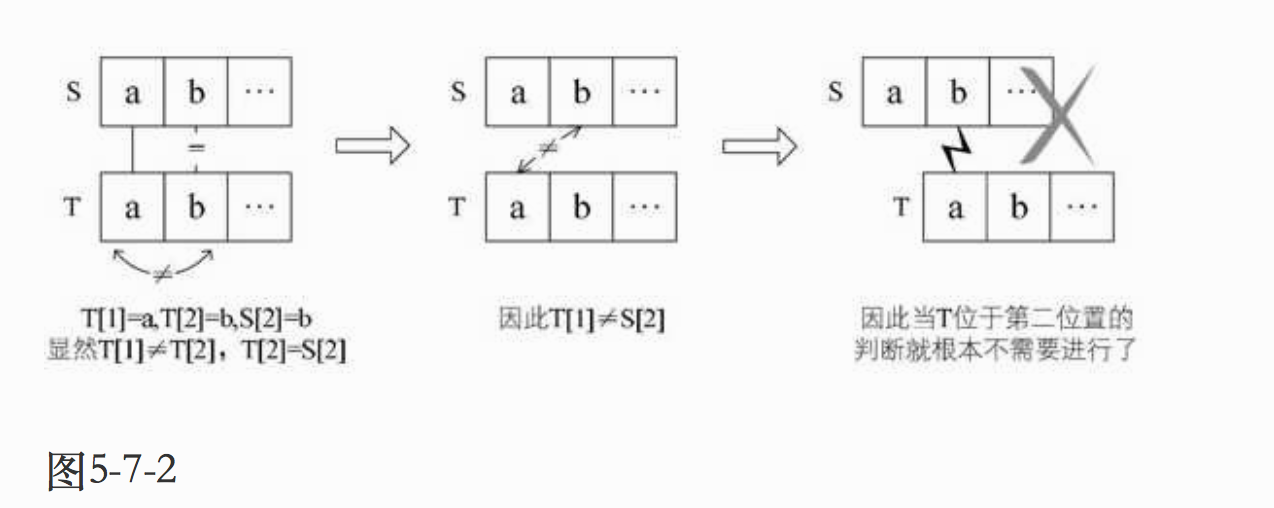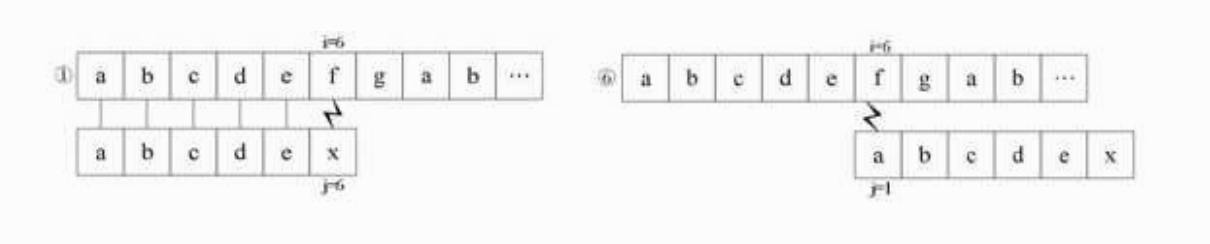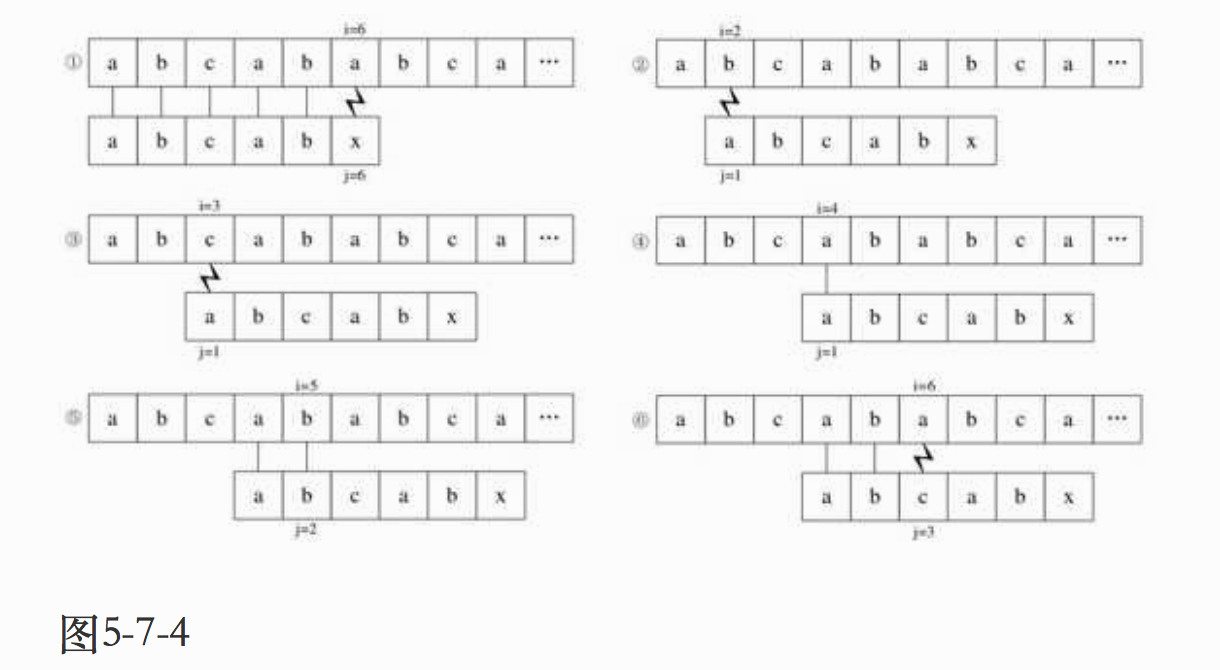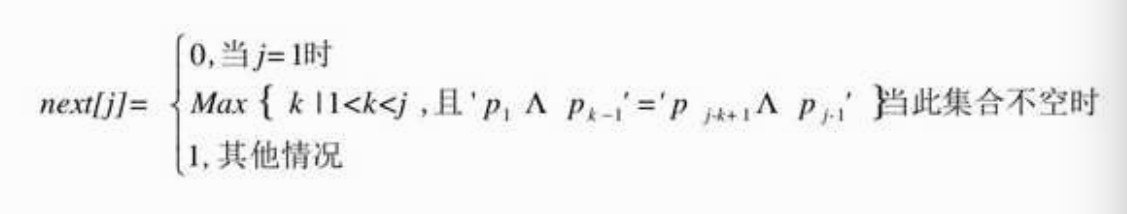# next数组推导

1．T="abcdex"

j          123456

next[j]        011111
1）当j=1时，next=0；

2）当j=2时，j由1到j-1就只有字符“a”，属于其他情况next=1；

3）当j=3时，j由1到j-1串是“ab”，显然“a”与“b”不相等，属其他情况，next=1；

4）以后同理，所以最终此T串的next[j]为011111。

2．T="abcabx"

j          123456

next[j]       011123
1）当j=1时，next=0；

2）当j=2时，同上例说明，next=1；

3）当j=3时，同上，next=1；

4）当j=4时，同上，next=1；

5）当j=5时，此时j由1到j-1的串是“abca”，前缀字符“a”与后缀字符“a”相等（前缀用下划线表示，后缀用斜体表示），因此可推算出k值为2（由‘p1...pk-1’=‘pj-k＋1...pj-1’，得到p1=p4）因此next=2；

6）当j=6时，j由1到j-1的串是“abcab”，由于前缀字符“ab”与后缀“ab”相等，所以next=3。

4．T="aaaaaaaab"

j          123456789

next[j]       012345678

1）当j=1时，next=0；

2）当j=2时，同上next=1；

3）当j=3时，j由1到j-1的串是“aa”，前缀字符“a”与后缀字符“a”相等，next=2；

4）当j=4时，j由1到j-1的串是“aaa”，由于前缀字符“aa”与后缀“aa”相等，所以next=3；

5）……

6）当j=9时，j由1到j-1的串是“aaaaaaaa”，由于前缀字符“aaaaaaa”与后缀“aaaaaaa”相等，所以next=8。

# KMP算法的优化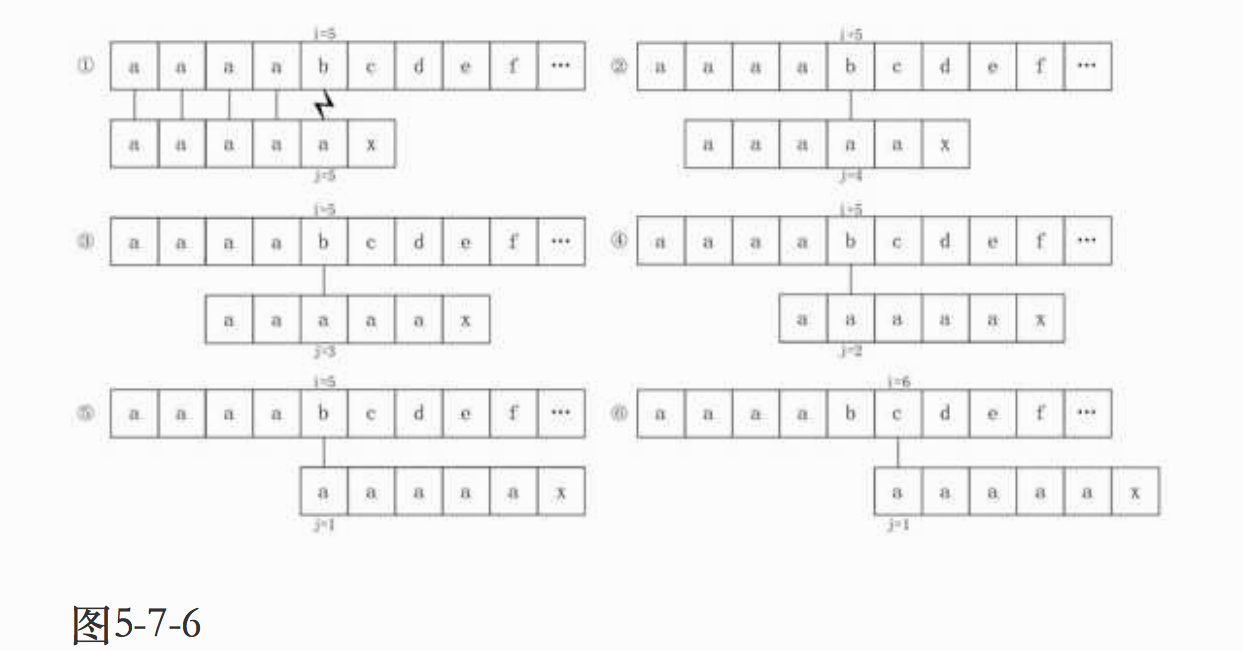# nextval数组值推导

1．T="ababaaaba"

j             123456789

next[j]          011234223
nextval[j]     010104210

1）当j=1时，nextval=0；

2）当j=2时，因第二位字符“b”的next值是1，而第一位就是“a”，它们不相等，所以nextval=next=1，维持原值。

3）当j=3时，因为第三位字符“a”的next值为1，所以与第一位的“a”比较得知它们相等，所以nextval=nextval=0；如图5-7-7所示。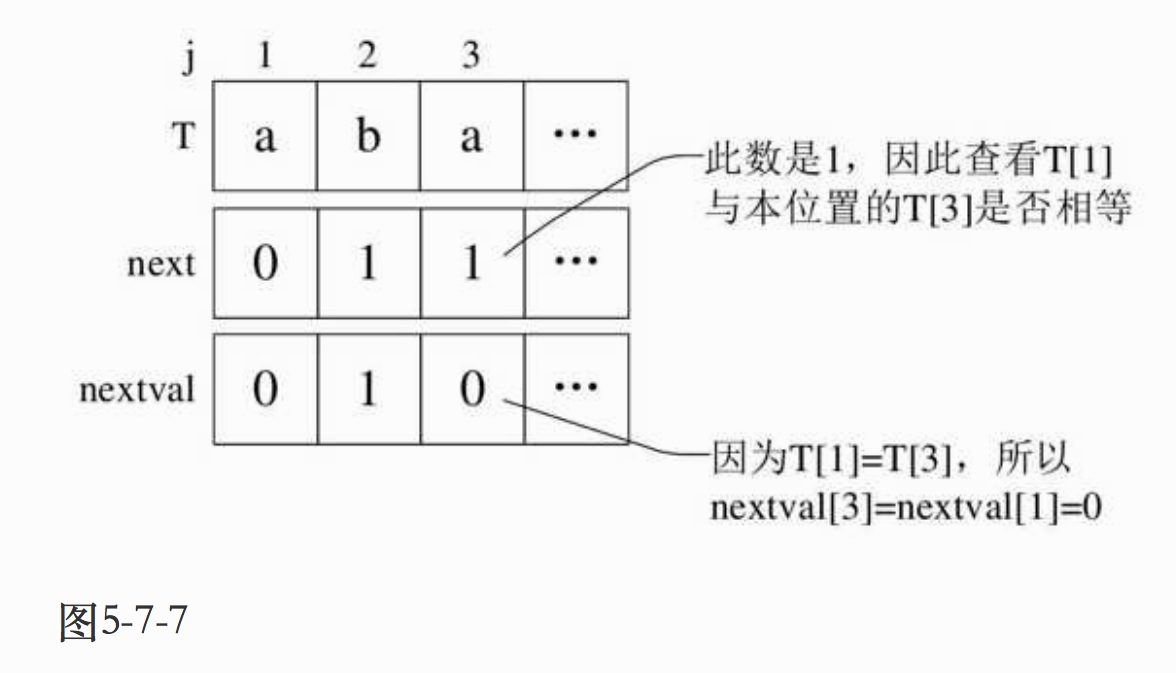4）当j=4时，第四位的字符“b”next值为2，所以与第二位的“b”相比较得到结果是相等，因此nextval=nextval=1；如图5-7-8所示。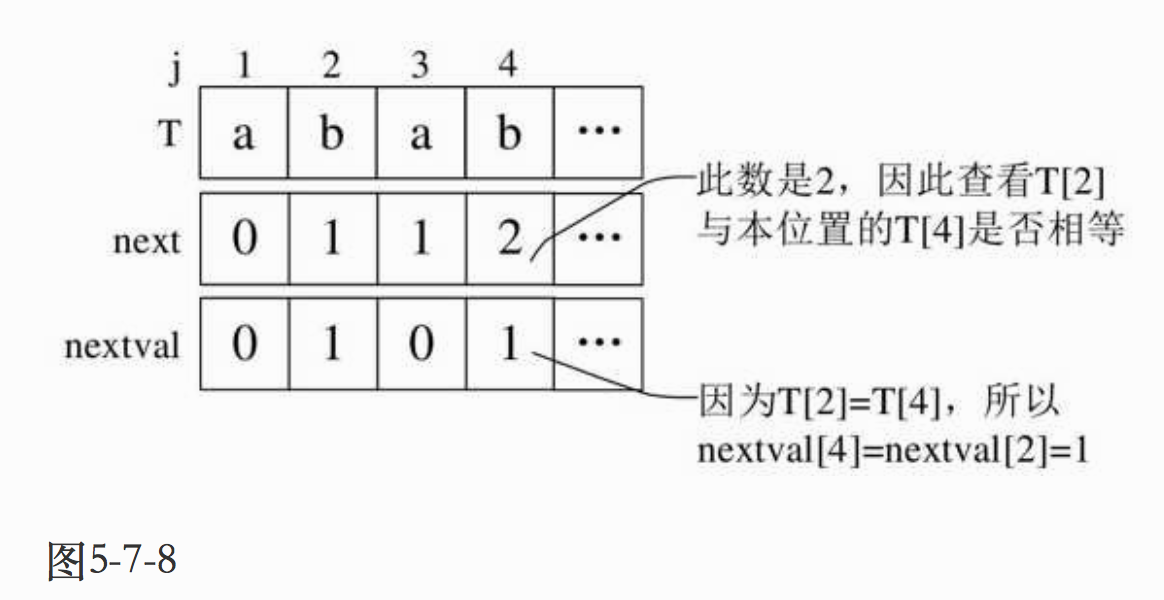5）当j=5时，next值为3，第五个字符“a”与第三个字符“a”相等，因此nextval=nextval=0；

6）当j=6时，next值为4，第六个字符“a”与第四个字符“b”不相等，因此nextval=4；

7）当j=7时，next值为2，第七个字符“a”与第二个字符“b”不相等，因此nextval=2；

8）当j=8时，next值为2，第八个字符“b”与第二个字符“b”相等，因此nextval=nextval=1；

9）当j=9时，next值为3，第九个字符“a”与第三个字符“a”相等，因此nextval=nextval=0。

2．T="aaaaaaaab"

j           123456789

next[j]        012345678
nextval[j]    000000008

1）当j=1时，nextval=0；

2）当j=2时，next值为1，第二个字符与第一个字符相等，所以nextval=nextval=0；

3）同样的道理，其后都为0……；

4）当j=9时，next值为8，第九个字符“b”与第八个字符“a”不相等，所以nextval=8。©️2019 CSDN 皮肤主题: 创作都市 设计师: CSDN官方博客